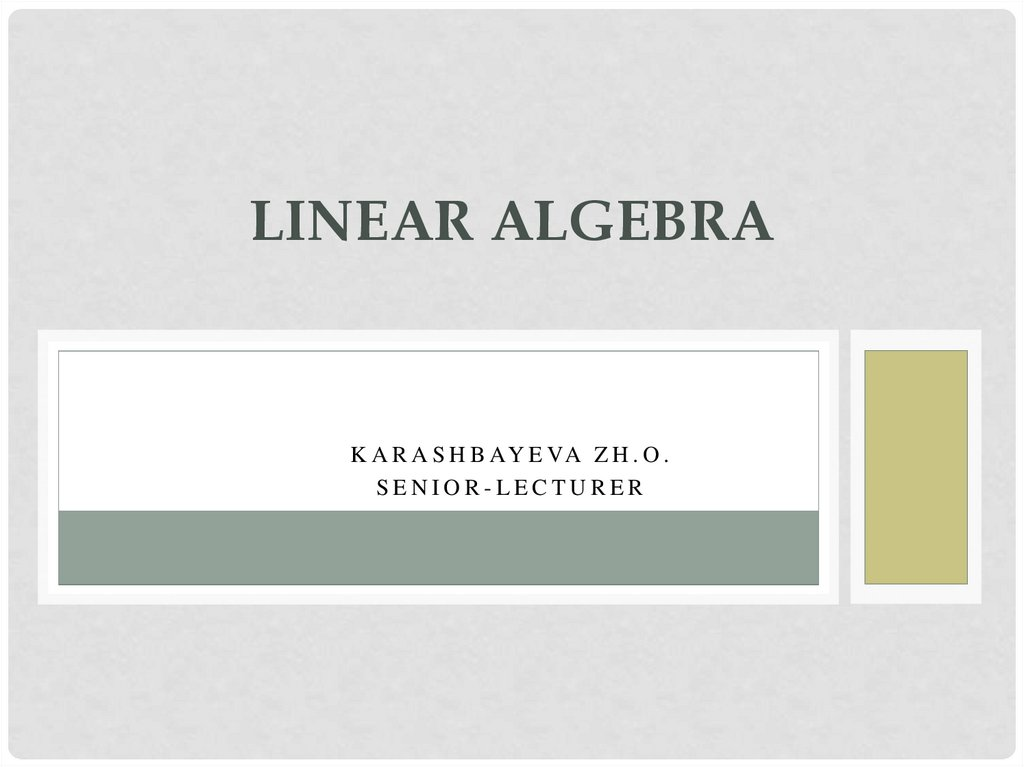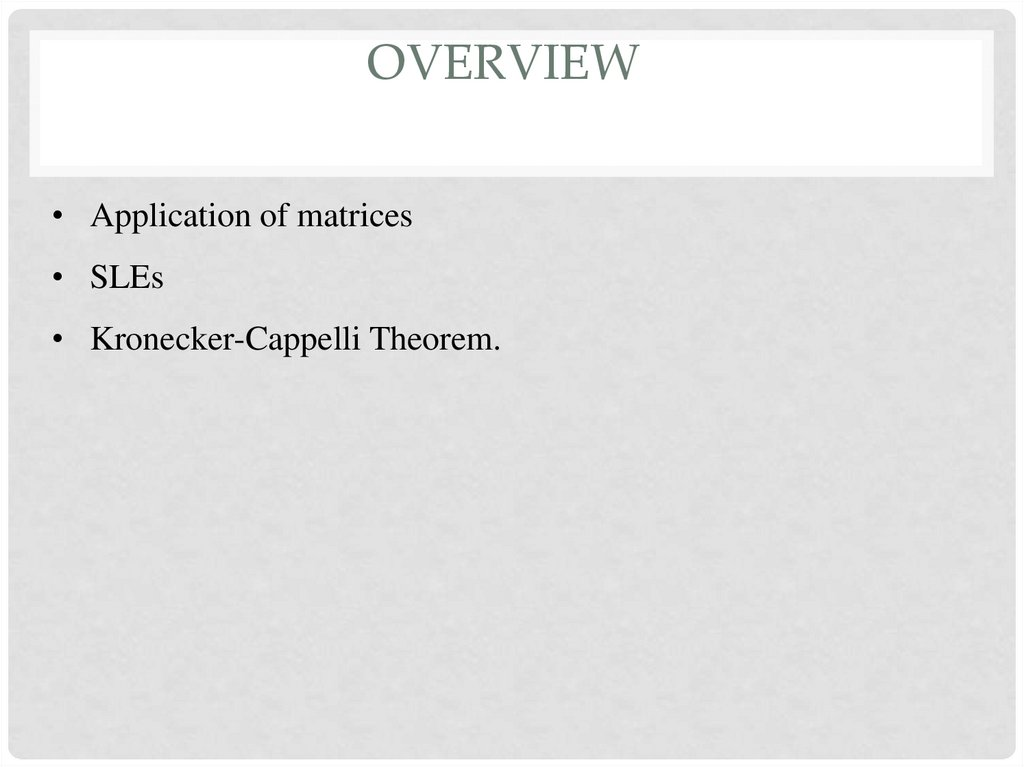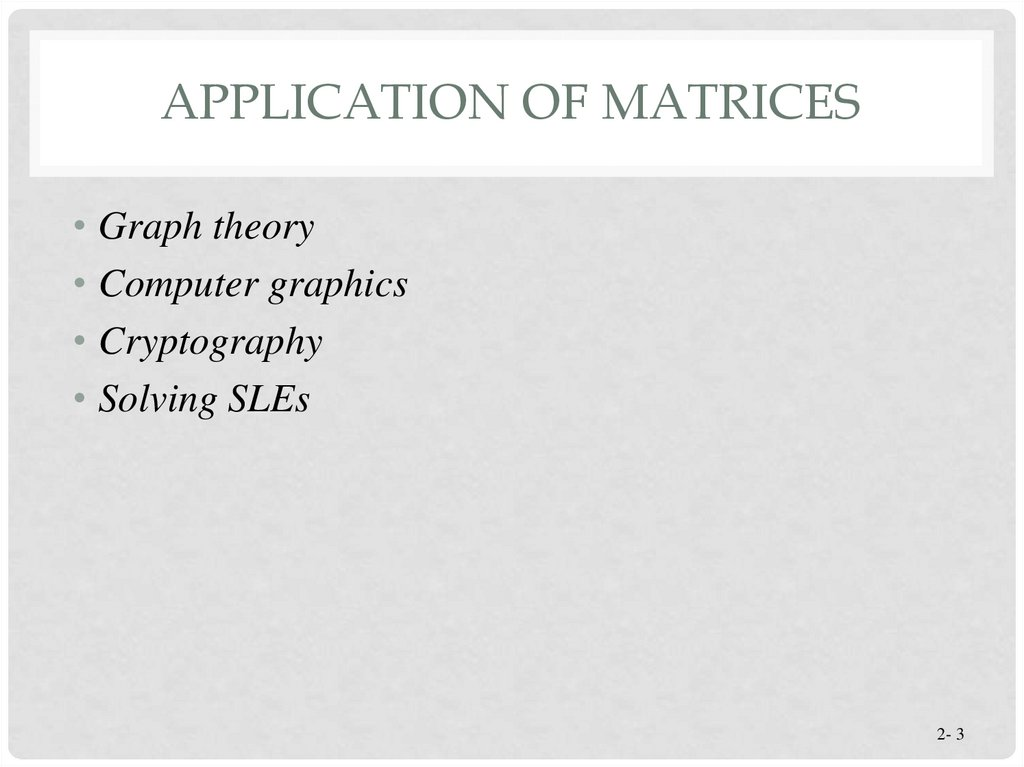# Linear Algebra. Lecture 5

## 1. Linear Algebra

LINEAR ALGEBRA
K A R A S H B AY E VA Z H . O .
SENIOR-LECTURER

## 2. overview

OVERVIEW
• Application of matrices
• SLEs
• Kronecker-Cappelli Theorem.

## 3. Application of matrices

APPLICATION OF MATRICES
• Graph theory
• Computer graphics
• Cryptography
• Solving SLEs
2- 3

GRAPH THEORY

## 5. Computer graphics

COMPUTER GRAPHICS

## 6. Computer graphics

COMPUTER GRAPHICS

## 7. Computer graphics

COMPUTER GRAPHICS

## 8. Cryptography

CRYPTOGRAPHY
• Study of encoding and decoding
secret messages
• Useful in sending sensitive information
so that only the intended receivers
can understand the message
• A common use of cryptography is to
send government secrets.

## 9. Encoding

A1
B2
C3
D4
E5
F6
G7
H8
I9
J 10
K 11
L 12
M 13
N 14
O 15
P 16
Q 17
ENCODING
R 18
S 19
• First we will assign numbers to
represent each letter of the alphabet. T 20
U 21
• We then create a “plaintext” matrix V 22
that holds the message in terms of
W 23
numbers.
X 24
Y 25
• Then we pick an invertible square
matrix, which can be multiplied with Z 26
_ 27
the “plaintext matrix”.
‘ 28

## 10.

1 2 1
2
2
4
1 3 3
Encrypting the Message
x
6 18 5 5 27 12 1 21 14 4 18 25 27
13 15 14 5 25 27 21 14 4 5 18 27 19
15 13 5 15 14 5 28 19 27 4 5 19 11
Encoding matrix
=
Plaintext
17 35 28 0
63 61 15 30 5 10 49 60 54
98 118 58 80 160 98 156 146 144 34 92 180 136
0 24 32 25 60 78 20 6 55 7 57 49 51
Ciphertext

## 11. Deciphering the Message

DECIPHERING THE MESSAGE
• In order to decode the message, we would have to take
the inverse of the encoding matrix to obtain the decoding
matrix.
• Multiplying the decoding matrix with the ciphertext would
result in the plaintext version.
• Then the arbitrarily assigned number scheme can be used
to retrieve the message.

## 12.

A1
B2
C3
D4
E5
F6
G7
H8
I9
J 10
K 11
L 12
M 13
N 14
Decrypting the Message
9 3
5
2
3
5 1
2 1
2 1
x
17 35 28 0
63 61 15 30 5 10 49 60
98 118 58 80 160 98 156 146 144 34 92 180
0 24 32 25 60 78 20 6 55 7 57 49
=
6 18 5 5 27 12 1 21 14 4 18 25
13 15 14 5 25 27 21 14 4 5 18 27
15 13 5 15 14 5 28 19 27 4 5 19
O 15
P 16
Q 17
R 18
S 19
T 20
U 21
54
V 22
136
W 23
51
X 24
Y 25
27
Z 26
19 _ 27
11
‘ 28

## 13.

YOU MIGHT WANT TO READ THIS.
A1
B2
C3
D4
E5
F6
G7
H8
I9
J 10
K 11
L 12
M 13
N 14
F
R
E
E
_
L
A
U
6
18
5
5
27 12
1
21 14
4 18 25 27
M
O
N
E
Y
U
N
D
E
13 15 14
5
25 27 21 14
4
5 18 27 19
O
_
D
E
S
28 19 27
4
5
19 11
_
M
E
O
N
E
15 13
5
15 14
5
'
S
N
D
R
R
Y
_
_
S
K
O 15
P 16
Q 17
R 18
S 19
T 20
U 21
V 22
W 23
X 24
Y 25
Z 26
_ 27
‘ 28

SOLVING SLES
2- 14

## 15. Kronecker-Cappelli Theorem

KRONECKER-CAPPELLI THEOREM
• Kronecker-Cappelli Theorem. A linear system has solutions
if and only if the rank of the matrix of the system A is equal
with the rank of the augmented matrix A’.
• 1. If rk(A) != rk(A’), a linear system is inconsistent (it doesn’t
have a solution)
• 2. If rk(A) = rk(A’) < n, a linear system has infinite solution
• 3. If rk(A) = rk(A’) = n, a linear system has only one solution

## 16.

Systems of Linear Equations
Graphing a system of two linear equations in two
unknowns gives one of three possible situations:
Case 1: Lines intersecting in a
single point. The ordered pair
that represents this point is the
unique solution for the system.
Section 8.1, Slide 16

## 17.

Systems of Linear Equations
Case 2: Lines that are distinct parallel
lines and therefore don’t intersect at
all. Because the lines have no common
points, this means that the system has
no solutions.
Section 8.1, Slide 17

## 18.

Systems of Linear Equations
Case 3: Two lines that are the
same line. The lines have an infinite
number of points in common, so
the system will have an infinite
number of solutions.